## 2014年6月3日 星期二

### Procedural Blocks: begin-end and fork-join

Procedural Blocks: begin-end and fork-join
begin-end and fork-join are used to combine a group of statements in a single block.
General syntax with begin-end is as follows:
 ```type_of_block @(sensitivity_list) begin: group_name local_variable_declarations; statements; end```

type_of_block may be initial or alwayssensitivity_list is optional and possible only in always block. You are knowing about initial and always block in the previous chapter.
Sensitivity list is used to model sequential or combinational logic. To make it more clear, observe these examples:
 ```module comb(a, b, c); input c; input b; output a; reg a; always @( c or b) begin a = c & b; end endmodule``` ```module seq(a, b, c); output a; input b; input c; reg a; always @( posedge c) begin a <= b; end endmodule```

 `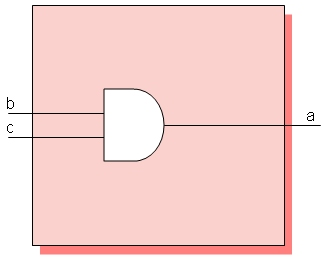` `Output of module comb` `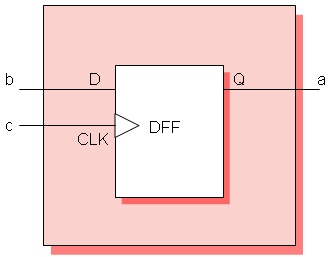` `Output of the module seq`
Inside an initial or always block, we can group statements using begin--end or fork--join.
These are some examples to understand how begin--end and fork--join executes.

 ```module forkjoin(clk, a, b); input clk; output a; output b; reg a, b; initial begin a = 0; b = 0; end always @(posedge clk) fork #2 a = 1; #1 b = 1; join endmodule``` ```module forkjoin1(clk, a, b); input clk; output a; output b; reg a, b; initial begin a = 0; b = 0; end always @(posedge clk) fork #2 a = 1; #1 b = a; join endmodule```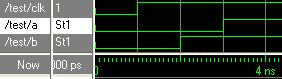Output of forkjoin ^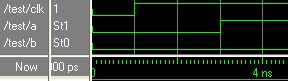Output of forkjoin1 ^ module beginend(clk, a,b);    input clk;    output a;    output b;    reg a, b;    initial    begin       a = 0;       b = 0;    end    always @(posedgeclk)    begin        #2 a = 1;        #1 b = a;    endendmodule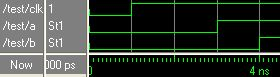Output of beginend ^
From these examples, you can understand the execution.
We can nest begin--end and fork--join. That is, inside a begin--end we can have fork--join and inside fork--join we can have begin--end.  Consider these codes to find out how nested begin--end and fork--join works.
 ```module nesting1(clk, a, b, c, d, e, f); input clk; output a, b, c, d, e, f; reg a, b, c, d, e, f; initial begin a = 0; b = 0; c = 0; d = 0; e = 0; f = 0; end always @(posedge clk) fork #2 a = 1; #2 b = 1; begin #2 c = 1; #2 d = 1; #2 e = 1; end #2 f = 1; join endmodule``` and here is the output: You can notice that a, b, c and f became high 2 ns after the clock, d 2ns after c and e 2ns after d.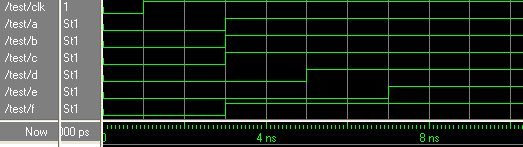module nesting2(clk, a, b, c, d, e, f);    input clk;    output a, b, c, d, e, f;    reg a, b, c, d, e, f;    initial    begin       a = 0;       b = 0;       c = 0;       d = 0;       e = 0;       f = 0;    end    always @(posedge clk)    begin        #2 a = 1;        #2 b = 1;        fork            #2 c = 1;            #2 d = 1;            #2 e = 1;        join        #2 f = 1;    endendmodule Output wave of the above code is here. Notice that a, b and c became 1 with 2ns delay in-between, d and e became 1 together with c, after that f with 2 ns delay.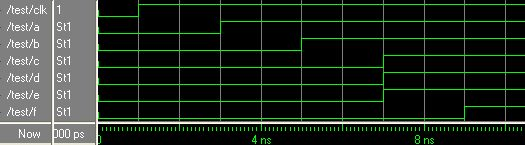Program control will come out of the fork--join block when all the statements finishes executing. in the case of code bellow, control will come out of block after 30ns.
fork
#20 c = 1;
#30 d = 1;
#10 e = 1;
join